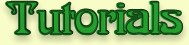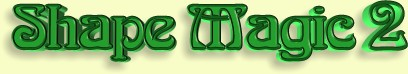Illustrated Guide to Special Effects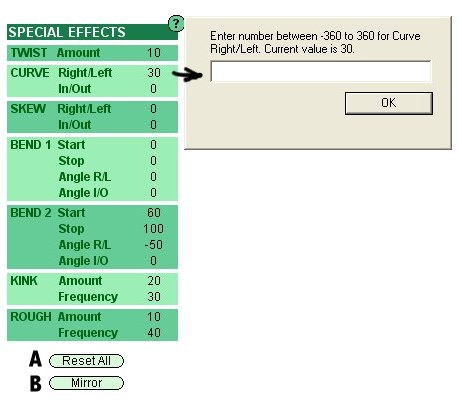On the left hand side of the Create File page is the Special Effects section. Values are entered by clicking on a number, which then brings up a dialog box. For example, clicking on 30 in the image brings up the dialog box for Curve Right/Left. The dialog box tells you what range of values can be used and what the current value is. If you click OK without entering a new value, or you enter a value outside the range, the current value is retained. Values can be reset to zero by typing in zero. An easier way to do this is to click on the Reset All button (A). Sometimes you will want to make a mirror model (like for animal horns). Click on the Mirror button (B) to change all angle values to their opposite value (e.g., 360 goes to -360). Note: depending upon the model, a mirror image may not have all angles the opposite.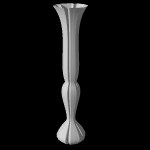No Effects: All the settings are 0. Click on the Reset All button to set all controls to zero.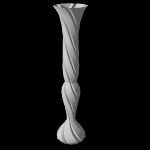Twist: Setting was 360 degrees. This means the cross-section rotates one complete turn from top to bottom. Enter a negative number to twist in the opposite direction. Numbers between -1440 and 1440 can be used.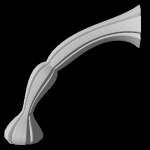Curve: Right/Left setting was 90 degrees. In/Out setting was 0 degrees. This means the model will evenly curve 90 degrees to the right from top to bottom. Enter a negative number to curve in the opposite direction. You can simultaneously curve Right/Left and In/Out. Numbers between -360 and 360 can be used.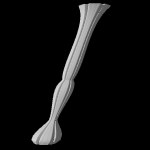Skew: Right/Left setting was 20 degrees. In/Out setting was 0 degrees. This means the model will evenly skew (displace the center line) 20 degrees to the right from top to bottom. Enter a negative number to skew in the opposite direction. You can simultaneously skew Right/Left and In/Out. Numbers between -80 and 80 can be used. Skew tends to lengthen the model - if this is a problem, shorten it by clicking on the icons at the bottom of the Side View page.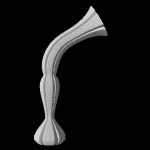Bend1: Start setting was 50% and Stop was 75%. Angle R/L (Right/Left) was 60 degrees and Angle I/O (In/Out) was 0 degrees. This means the model will evenly bend 60 degrees starting 50% up the model and stopping 75% up the model. Enter a negative number to curve in the opposite direction. You can simultaneously bend Right/Left and In/Out. Angles between -360 and 360 can be used.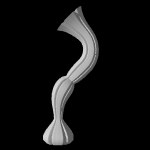Bend2: This shows both Bend1 and Bend2 in use. Bend1 Start setting was 25% and Stop was 45%. Angle R/L (Right/Left) was 45 degrees and Angle I/O (In/Out) was 0 degrees. Bend2 Start setting was 60% and Stop was 85%. Angle R/L (Right/Left) was -90 degrees and Angle I/O (In/Out) was 0 degrees.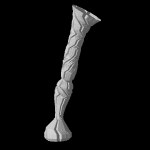Kink: Kink randomly tilts the cross-section each time it is drawn. This can give a more natural look to items like tree trunks and stems. Amount setting was 50 and Frequency was 50%. A larger Amount setting makes for greater tilting. Frequency is the % of time the cross-section is tilted. Amount can range between 0 and 50. Frequency can range between 0 and 100%. The amount of kink is also affected by the number of bezier segments in the Side View. A larger number of bezier segments gives more kink.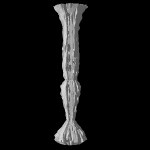Rough: Rough randomly expands or shrinks the surface of the model. This can give a more natural look to items like tree trunks, flowers and stones. Amount setting was 20% and Frequency was 25%. A larger Amount setting makes for greater roughness. Frequency is the % of time the roughness is applied to each Top View bezier segments. Amount can range between 0 and 100%. Frequency can range between 0 and 100%. The amount of roughness is also affected by the number of bezier segments in the Side View and Top View. A larger number of bezier segments give more, but smaller, roughness.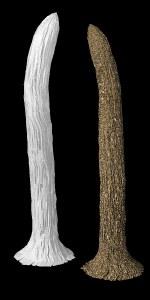Combinations: Special Effects can be combined as shown by this tree trunk. Cross-section was a Smooth Petal with 7 petals and 8 bezier segments. Twist was 10 degrees. Curve Right/Left was 30 degrees. Bend1 Start was 60%, Stop was 100% and Angle R/L was -50 degrees. Kink Amount was 20 and Frequency was 30%. Roughness Amount was 10% and Frequency was 40%.Combinations: Special Effects can be combined as shown by these animal horns. Cross-section was Round. Twist was 500 degrees and Curve Right/Left was 360 degrees. The second horn was made by clicking on the Mirror button at the bottom of the Special Effects section.